Most Affordable JEE | NEET | 8,9,10 Preparation by Kota's Top IITian Doctor Faculties

# Properties of Diamagnetic Materials - Magnetism & Matters | Class 12 Physics NotesHere we will study about the Paramagnetic and Diamagnetic Materials. Properties of Diamagnetic Materials & Paramagnetic Substances also discussed in this article.

### Diamagnetic Materials

1. The substances which when placed in a magnetising field get feebly magnetised in a direction opposite to magnetising field are called diamagnetic.2. The substances are weakly repelled by the field so in a non uniform field these have a tendency to move from strong to weak field.
3. A diamagnetic rod sets itself perpendicular to field because field is strongest at poles.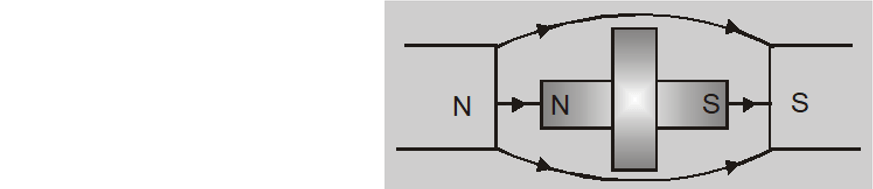4. A diamagnetic liquid in a U-tube depresses in the limb which is between the poles of magnet.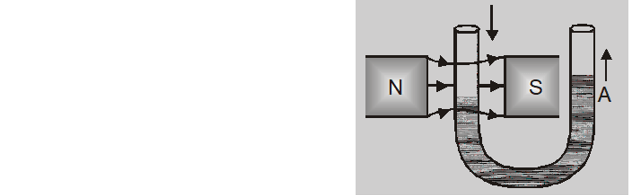5. Intensity of magnetisation I is very small, negative and proportional to magnetising field.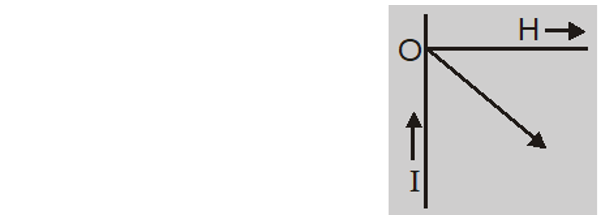6. Magnetic susceptibility $\chi_{\mathrm{m}}=\frac{\mathrm{I}}{\mathrm{H}}$ is small and negative $\left(\approx 10^{-5}\right)$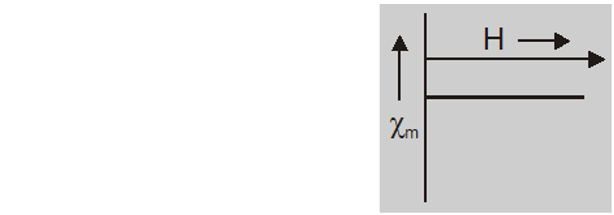7. The relative permeability $\mu_{\mathrm{r}}=\frac{\mu}{\mu_{0}}$ is slightly less than unity.
8. The field inside the material $\mathrm{B}$ is less than magnetising field H. They have a tendency to expel lines of force.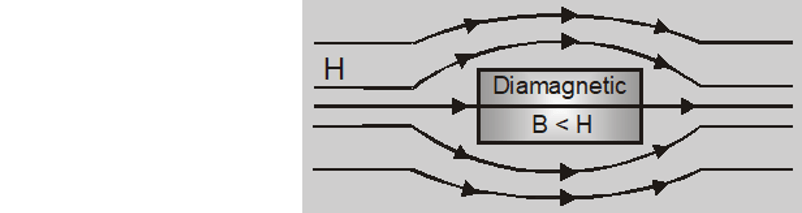9. Magnetic dipole moment (M) is small and opposite to magnetising field H.
10. Diamagnetic substances do not obey Curie's law and show no transition
11. at Curie temperature $X_{m}$ is indepedent of temperature.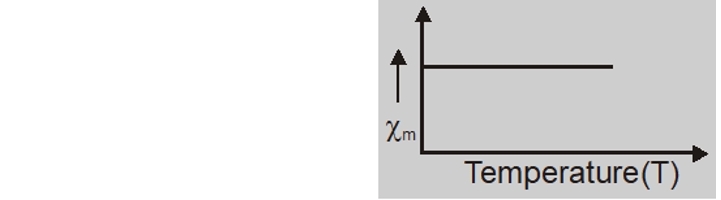12. The origin of diamagnetism is the induced dipole moment due to change in orbital motion of electrons in atoms by applied field.
13. Imp. Diamagnetism is present in all materials and is independent of temperature. As it is weak it is often masked by para and ferromagnetic effects.
14. The atoms do not have any permanent dipole moment i.e. paired spin.
15. Diamagnetism is exhibited by solids, liquids and gases.
16. If a diamagnetic liquid is placed in a watch glass placed. on two pole pieces which are quite close to each other then liquid accumulates at sides and shows depression in the middle, where field is strongest.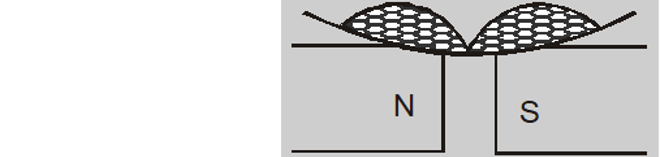17. If a diamagnetic liquid is placed in a watch glass placed on two pole pieces which are sufficiently apart then liquid accumulates in the middle where field is weakest.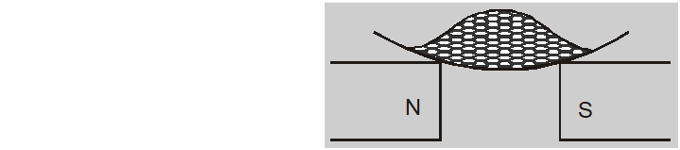Diamagnetic substances are $\mathrm{cu}, \mathrm{zn}, \mathrm{Bi}, \mathrm{Ag},$ Au, Pb, He, $\mathrm{Ar}, \mathrm{NaC} \ell, \mathrm{H}_{2} \mathrm{O},$ marble,glass etc.     Click here for the Video tutorials of Magnetic Effect of Current Class 12
About eSaral At eSaral we are offering a complete platform for IIT-JEE & NEET preparation. The main mission behind eSaral is to provide education to each and every student in India by eliminating the Geographic and Economic factors, as a nation’s progress and development depends on the availability of quality education to each and every one. With the blend of education & technology, eSaral team made the learning personalized & adaptive for everyone.